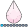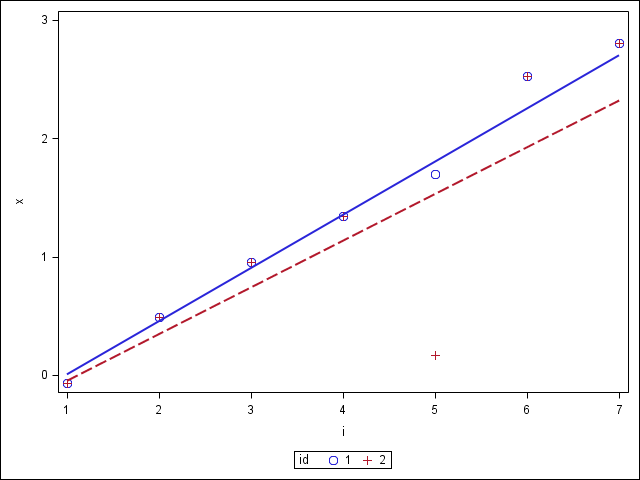NKormanik
Barite | Level 11

## Regression-type problem, data in a row...

Data lie in a row, not a column.  In SAS dataset.  From SAS output.  Would prefer to use SAS, if possible.

Assume series 1, 2, 3, 4, 5, in single row, across.

What comes next?

6, of course.

What if data are more messy?  How to project that??

Somewhat like a time series problem.

Prefer answer be in same row as data.  Just to the right, in it's own column.

Willing to use another program, such as Excel, if SAS can't do it.

Any help appreciated.

Nicholas

1 ACCEPTED SOLUTION

Accepted Solutions

## Re: Regression-type problem, data in a row...

Number of rows is not relevant. I mixed up x/y initially.

If you have a SAS/IML license I'm sure it's much easier in SAS IML.

Formula courtesy of google + verification with proc reg.

http://www4.stat.ncsu.edu/~dickey/Summer_Institute/formulas.pdf

You'll need to figure out a way to get your X array properly declared, but this should get you started.

Alternatively you could use a formula that doesn't depend on an X array. I suggest trying it yourself, but in case you want, that solution is also below, hidden.

http://www.statisticshowto.com/how-to-find-a-linear-regression-slope/

``````
data test;
input x1 x2 x3 x4 x5 x6;
datalines;
-0.069965723 0.492749371 0.955245597 1.346963522 1.701118239 2.523141195
;
run;

data slope;
set test;
array ys(6) x1-x6;
array vals(6) (1 2 3 4 5 6);
xbar = mean(of vals(*));
ybar = mean(of ys(*));

do i=1 to dim(vals);

num=sum(num, (vals(i)-xbar)*(ys(i)-ybar));
den=sum(den, (vals(i)-xbar)**2);

end;

slope = num/den;
run;

proc transpose data=test out=test2(rename=col1=y);
run;

data test2;
set test2;
x=_n_;
run;

proc reg data=test2;
model y=x;
run;
``````

Without x array:

Spoiler
16024 data slope;
16025 set test;
16026 array ys(6) x1-x6;
16027 array vals(6) (1 2 3 4 5 6);
16028 xbar = mean(of vals(*));
16029 ybar = mean(of ys(*));
16030
16031
16032
16033 do i=1 to dim(vals);
16034 s_xy=sum(s_xy, i*ys(i));
16035 s_y=sum(s_y, ys(i));
16036 s_x2=sum(s_x2, vals(i)**2);
16037
16038 num=(vals(i)-xbar)*(ys(i)-ybar);
16039 den=(vals(i)-xbar)**2;
16040
16041 num_tot=sum(num, num_tot);
16042 den_tot=sum(den, den_tot);
16043 end;
16044
16045 s_x=sum(of vals(*));
16046 n=dim(vals);
16047
16048 slope = (n*s_xy - s_x*s_y)/(n*s_x2 - s_x**2);
16049 slope2 = num_tot/den_tot;
16050 run;

12 REPLIES 12

## Re: Regression-type problem, data in a row...

Transpose to long, use proc reg and predict out and then transpose back?NKormanik
Barite | Level 11

## Re: Regression-type problem, data in a row...

Maybe have to do that.  Was hoping for some SAS procedure that will project out with data staying as is in row.

## Re: Regression-type problem, data in a row...

Not that I could think of. You could probably combine functions to do a linear regression across a row without too much difficulty, I think all of the functions are there.

If using Excel there's a LINEST function that can help.NKormanik
Barite | Level 11

## Re: Regression-type problem, data in a row...

"You could probably combine functions to do a linear regression across a row without too much difficulty, I think all of the functions are there."

All I really need is the slope for each series of numbers in the row.

``````_1	_2	_3	_4	_5	_8
-0.069965723	0.492749371	0.955245597	1.346963522	1.701118239	2.523141195
``````

I have thousands of such rows where the slope is needed.  Any help would be greatly appreciated.

A new column following the series should contain the slope value for that particular series.

Nicholas

## Re: Regression-type problem, data in a row...

Number of rows is not relevant. I mixed up x/y initially.

If you have a SAS/IML license I'm sure it's much easier in SAS IML.

Formula courtesy of google + verification with proc reg.

http://www4.stat.ncsu.edu/~dickey/Summer_Institute/formulas.pdf

You'll need to figure out a way to get your X array properly declared, but this should get you started.

Alternatively you could use a formula that doesn't depend on an X array. I suggest trying it yourself, but in case you want, that solution is also below, hidden.

http://www.statisticshowto.com/how-to-find-a-linear-regression-slope/

``````
data test;
input x1 x2 x3 x4 x5 x6;
datalines;
-0.069965723 0.492749371 0.955245597 1.346963522 1.701118239 2.523141195
;
run;

data slope;
set test;
array ys(6) x1-x6;
array vals(6) (1 2 3 4 5 6);
xbar = mean(of vals(*));
ybar = mean(of ys(*));

do i=1 to dim(vals);

num=sum(num, (vals(i)-xbar)*(ys(i)-ybar));
den=sum(den, (vals(i)-xbar)**2);

end;

slope = num/den;
run;

proc transpose data=test out=test2(rename=col1=y);
run;

data test2;
set test2;
x=_n_;
run;

proc reg data=test2;
model y=x;
run;
``````

Without x array:

Spoiler
16024 data slope;
16025 set test;
16026 array ys(6) x1-x6;
16027 array vals(6) (1 2 3 4 5 6);
16028 xbar = mean(of vals(*));
16029 ybar = mean(of ys(*));
16030
16031
16032
16033 do i=1 to dim(vals);
16034 s_xy=sum(s_xy, i*ys(i));
16035 s_y=sum(s_y, ys(i));
16036 s_x2=sum(s_x2, vals(i)**2);
16037
16038 num=(vals(i)-xbar)*(ys(i)-ybar);
16039 den=(vals(i)-xbar)**2;
16040
16041 num_tot=sum(num, num_tot);
16042 den_tot=sum(den, den_tot);
16043 end;
16044
16045 s_x=sum(of vals(*));
16046 n=dim(vals);
16047
16048 slope = (n*s_xy - s_x*s_y)/(n*s_x2 - s_x**2);
16049 slope2 = num_tot/den_tot;
16050 run;NKormanik
Barite | Level 11

## Re: Regression-type problem, data in a row...

"If you have a SAS/IML license I'm sure it's much easier in SAS IML."

Not sure is SAS/IML is available here.  Assuming that it is, would you, or someone, please post code which might work to get the slopes of the rows?

Thanks!

## Re: Regression-type problem, data in a row...

I don't code in IML.

Did the above solutions work?NKormanik
Barite | Level 11

## Re: Regression-type problem, data in a row...

How about THAT!  I used the code in your "Spoiler Allert" and it came up with the same values that Excel came up with.  Soooo..., you did it.

"Slope2" was the column with the same answers.  Not sure what "Slope" column was, or many of the other columns you created.

Before I mark this question as completed, do you want to explain anything further, or make any final changes?

Thank you very, very much Reeza.NKormanik
Barite | Level 11

## Re: Regression-type problem, data in a row...

Here is the actual code I used.  Changing ONLY the first three lines.  Leaving alone all the rest:

``````data nicholas.slope;
set nicholas.test;
array ys(6) _50501 _50502 _50503 _50504 _50505 _50508;
array vals(6) (1 2 3 4 5 8);
xbar = mean(of vals(*));
ybar = mean(of ys(*));

do i=1 to dim(vals);
s_xy=sum(s_xy, i*ys(i));
s_y=sum(s_y, ys(i));
s_x2=sum(s_x2, vals(i)**2);

num=(vals(i)-xbar)*(ys(i)-ybar);
den=(vals(i)-xbar)**2;

num_tot=sum(num, num_tot);
den_tot=sum(den, den_tot);
end;

s_x=sum(of vals(*));
n=dim(vals);

slope = (n*s_xy - s_x*s_y)/(n*s_x2 - s_x**2);
slope2 = num_tot/den_tot;
run;

``````PGStats
Opal | Level 21

## Re: Regression-type problem, data in a row...

For a more robust estimator of slope, you could use Sen's slope. Here is an illustration of the effect of having an outlier in your data (when id=2):

``````data test;
input id x1 x2 x3 x4 x5 x6;
datalines;
1 -0.069965723 0.492749371 0.955245597 1.346963522 1.701118239 2.523141195
2 -0.069965723 0.492749371 0.955245597 1.346963522 .1701118239 2.523141195
;

data want;
set test;
array _x x:;
array _p{100} _temporary_; /* Greater than dim(_x)*(dim(_x)-1)/2 */
array _s{100} _temporary_; /* Greater than dim(_x)*(dim(_x)-1)/2 */

call missing(of _p{*}, of _s{*});
k = dim(_x) + 1;
p = 0;
do i = 1 to dim(_x)-1;
do j = i+1 to dim(_x);
p + 1;
_p{p} = (_x{j}*(k-i) - _x{i}*(k-j))/(j-i);
_s{p} = (_x{j} - _x{i})/(j-i);
end;
end;
x_pred = median(of _p{*});
slope = median(of _s{*});
drop i j k p;
run;

proc print; run;

data graph;
set want;
array _x x:;
do i = 1 to dim(_x);
x = _x{i};
if i < dim(_x) then y = x;
output;
end;
keep id i x y;
run;

proc sgplot data=graph;
scatter x=i y=x / group=id markerattrs=(size=9);
reg     x=i y=y / group=id nomarkers;
run;``````
``` Obs  id      x1        x2       x3       x4       x5       x6     x_pred   slope

1    1  -0.069966  0.49275  0.95525  1.34696  1.70112  2.52314  2.80523  0.47231
2    2  -0.069966  0.49275  0.95525  1.34696  0.17011  2.52314  2.80523  0.47231
```PGNKormanik
Barite | Level 11

## Re: Regression-type problem, data in a row...

Not only another way of finding slope for values across a row, but a great contribution.

I'll try to incorporate your code, and create an additional column beside the existing slope column, to compare the two results.

Thank you very much, PGStats.  Wonderful to have you present and so active.

NicholasNKormanik
Barite | Level 11

## Re: Regression-type problem, data in a row...

PGStats, I see that you are comparing the two lines, demonstrating outlier influence, and I appreciate that.

But I'm not able to extract the alternative equation for slope, or way to actually calculate it.

Would you please simplify and provide the robust formula for slope (assuming we are working with rows, across, not columns)?

I presently have a column of slope measures using the formula given by Ksharp.  I'd like to add another column of slope measures next to it using the alternative robust formula you've introduced, to compare the two columns.

Thanks!

Discussion stats
• 12 replies
• 1993 views
• 3 likes
• 3 in conversation